Ex 9.2

Chapter 9 Class 11 Sequences and Series
Serial order wise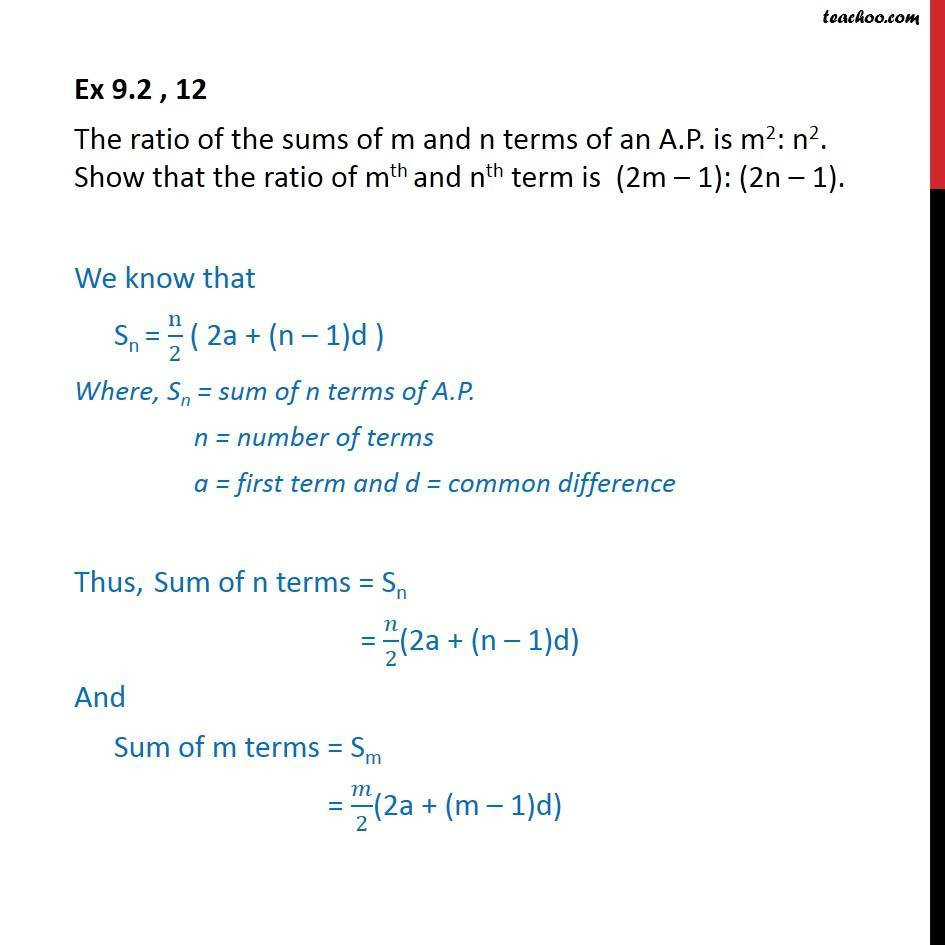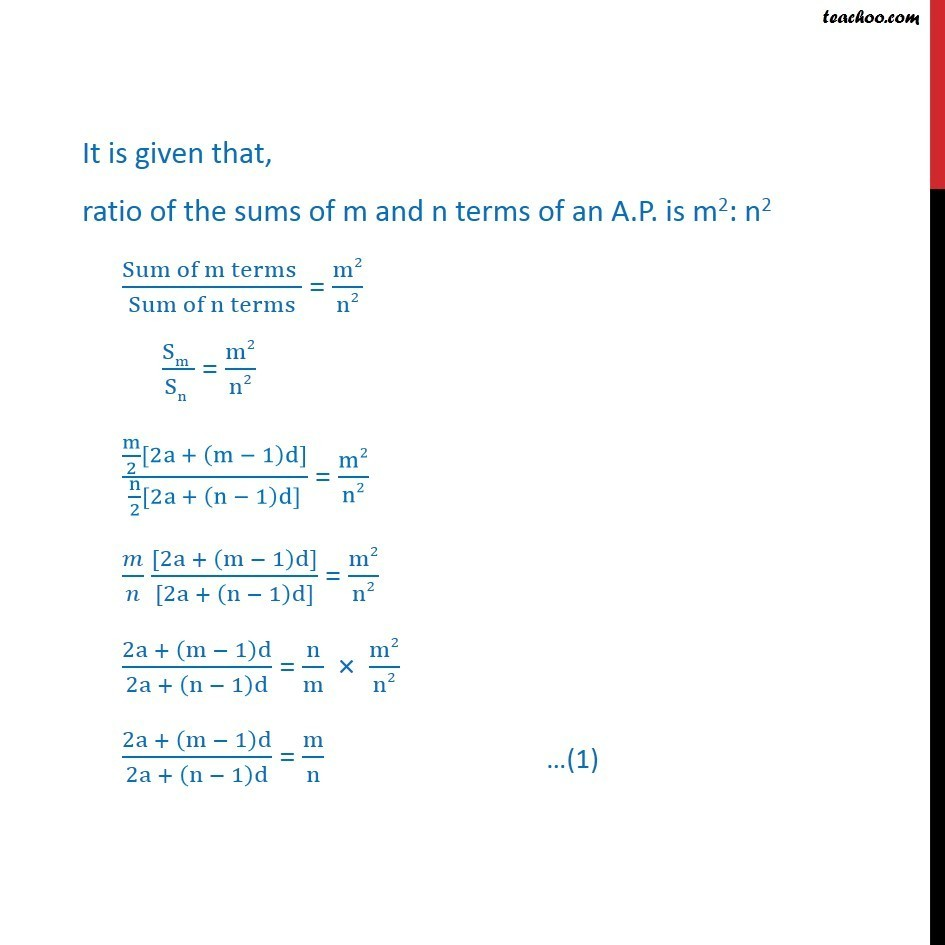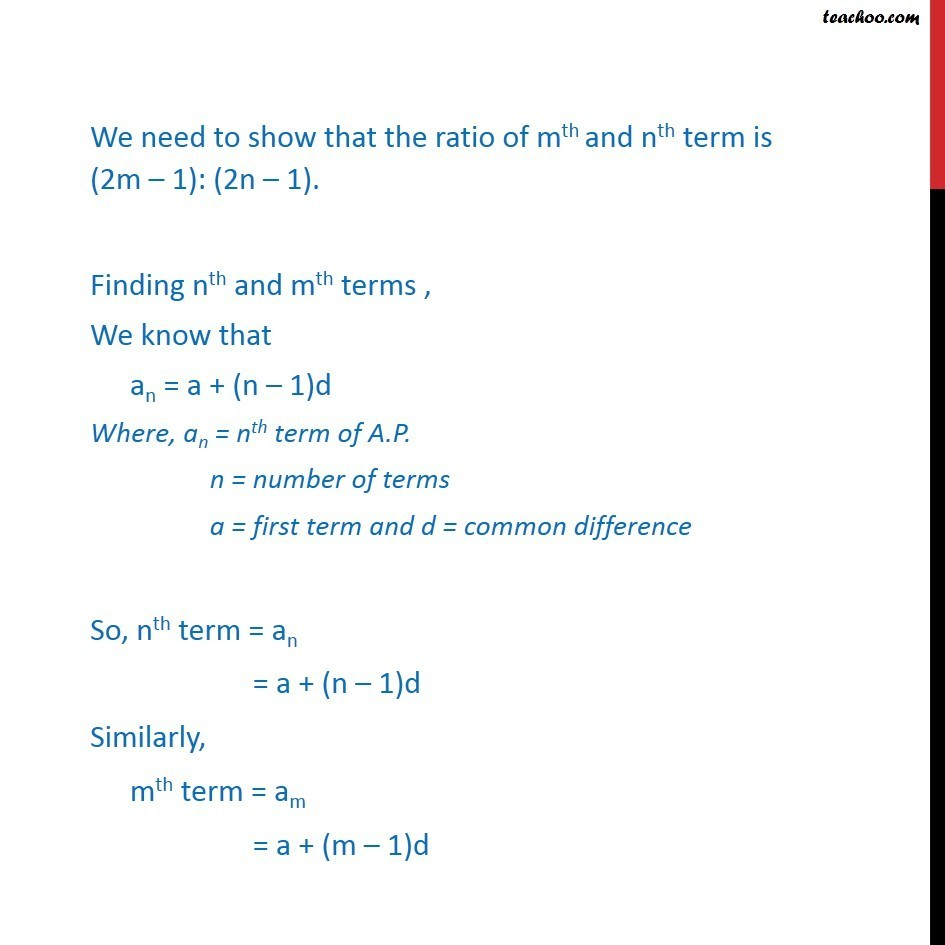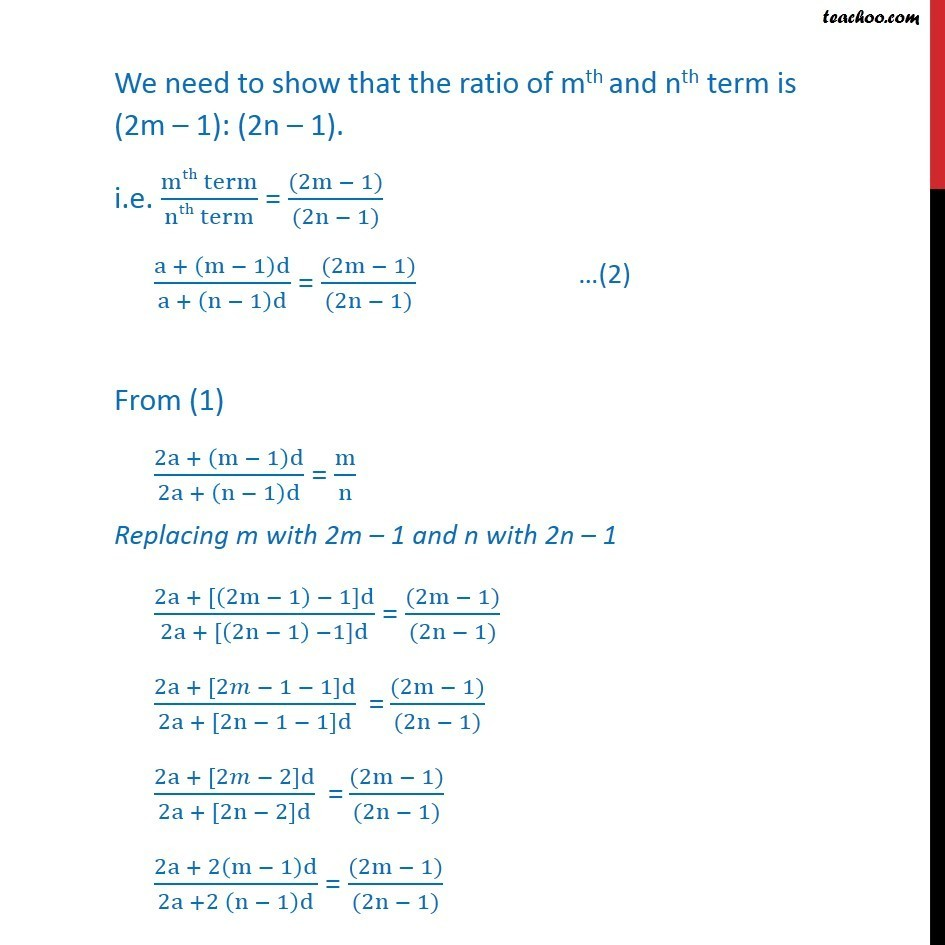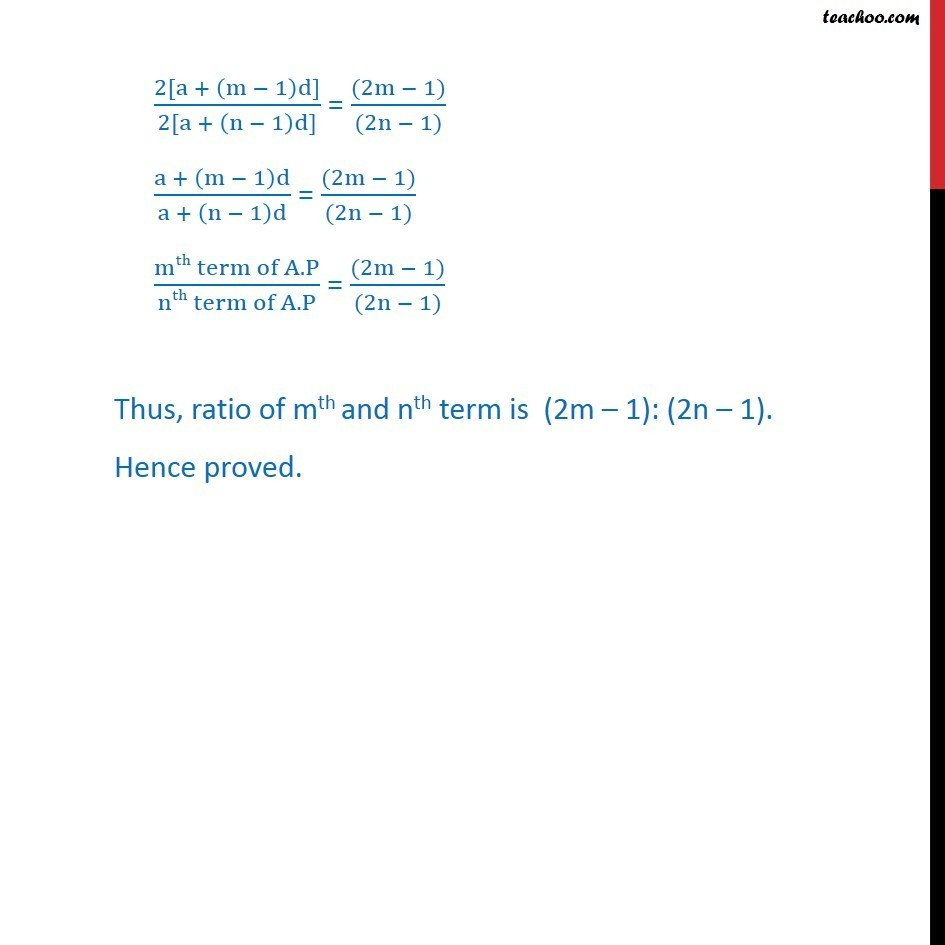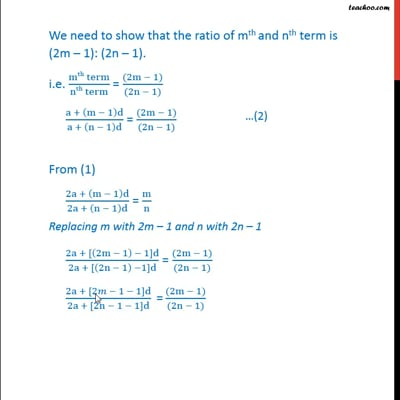This video is only available for Teachoo black users

Introducing your new favourite teacher - Teachoo Black, at only ₹83 per month

### Transcript

Ex 9.2 , 12 The ratio of the sums of m and n terms of an A.P. is m2: n2. Show that the ratio of mth and nth term is (2m 1): (2n 1). We know that Sn = n/2 ( 2a + (n 1)d ) Where, Sn = sum of n terms of A.P. n = number of terms a = first term and d = common difference Thus, Sum of n terms = Sn = /2(2a + (n 1)d) And Sum of m terms = Sm = /2(2a + (m 1)d) It is given that, ratio of the sums of m and n terms of an A.P. is m2: n2 (Sum of m terms )/(Sum of n terms) = m2/n2 (Sm )/(Sn ) = m2/n2 (m/2[2a + (m 1)d])/(n/2[2a + (n 1)d]) = m2/n2 / ([2a + (m 1)d])/([2a + (n 1)d]) = m2/n2 (2a + (m 1)d)/(2a + (n 1)d) = n/m m2/n2 (2a + (m 1)d)/(2a + (n 1)d) = m/n We need to show that the ratio of mth and nth term is (2m 1): (2n 1). Finding nth and mth terms , We know that an = a + (n 1)d Where, an = nth term of A.P. n = number of terms a = first term and d = common difference So, nth term = an = a + (n 1)d Similarly, mth term = am = a + (m 1)d We need to show that the ratio of mth and nth term is (2m 1): (2n 1). i.e. (mth term)/(nth term) = ((2m 1))/((2n 1)) (a + (m 1)d)/(a + (n 1)d) = ((2m 1))/((2n 1)) From (1) (2a + (m 1)d)/(2a + (n 1)d) = m/n Replacing m with 2m 1 and n with 2n 1 (2a + [(2m 1) 1]d)/(2a + [(2n 1) 1]d) = ((2m 1))/((2n 1)) (2a + [2 1 1]d)/(2a + [2n 1 1]d) " =" ((2m 1))/((2n 1)) (2a + [2 2]d)/(2a + [2n 2]d) " =" ((2m 1))/((2n 1)) (2a + 2(m 1)d)/(2a +2 (n 1)d) = ((2m 1))/((2n 1)) (2[a + (m 1)d])/(2[a + (n 1)d]) = ((2m 1))/((2n 1)) (a + (m 1)d)/(a + (n 1)d) = ((2m 1))/((2n 1)) (mth term of A.P)/(nth term of A.P) = ((2m 1))/((2n 1)) Thus, ratio of mth and nth term is (2m 1): (2n 1). Hence proved.